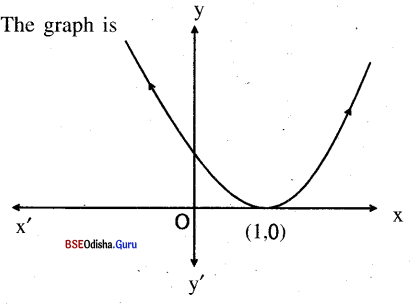# CHSE Odisha Class 11 Math Solutions Chapter 3 Relations And Function Ex 3(c)

Odisha State Board CHSE Odisha Class 11 Math Solutions Chapter 3 Relations And Functions Ex 3(c) Textbook Exercise Questions and Answers.

## CHSE Odisha Class 11 Math Solutions Chapter 3 Relations And Functions Exercise 3(c)

Question 1.
Give an example of a relation that is not a function.
Solution:
Let the relation ‘f’ be defined from
A = {1, 2, 3} to B = {1, 2} as
f = {(1, 1), (1, 2), (2, 1), (3, 2)}
f is not a function because f (1) = 1,
f(1) = 2 but 1 ± 2 i.e. f is one many.

Question 2.
If X and Y are sets containing m and n elements respectively then what is the total number of functions from X to Y?
Solution:
If |X| = m and |Y| = n then the number of functions from X to Y = |Y||X| = nmQuestion 3.
Find the domain of the following functions:
(i)$$\sqrt{9-x^2}$$
Solution:
y = f(x) = $$\sqrt{9-x^2}$$
Clearly, f(x) is well-defined for 9 – x2 ≥ 0 ⇒ x2 ≤ 32 ⇒ – 3 ≤ x ≤ 3
∴ dom f = [ – 3, 3]

(ii) $$\frac{x}{1+x}$$
Solution:
y = f(x) = $$\frac{x}{1+x}$$
f(x) is well defined for all x ∈ R
∴ dom f = R

(iii) 1 – |x|
Solution:
y = f(x) = 1 – |x|
f(x) is well defined for all x ∈ R
∴ dom f = R

(iv) $$\frac{1}{x^2-1}$$
Solution:
y = f(x) = $$\frac{1}{x^2-1}$$
f(x) is well defined for x2 – 1 ≠ 0
⇒ x2 ≠ 1
⇒ x2 ≠ ± 1
∴ dom f = R – { -1, 1}

(v) $$\frac{\sin x}{1+\tan x}$$
Solution:
y – f(x) = $$\frac{\sin x}{1+\tan x}$$
f(x) is well defined for 1 + tan x
≠ 0
⇒ tan x ≠ – 1
⇒ x ≠ n π + $$\frac{3 \pi}{4}$$
∴ dom f = R – {n π + $$\frac{3 \pi}{4}$$}

(vi) $$\frac{x}{|x|}$$
Solution:
y = f(x) = $$\frac{x}{|x|}$$
f(x) is well defined for |x| ≠ 0
⇒ x ≠ 0
∴ dom f = R – {0}

(vii) $$\frac{1}{x+|x|}$$
Solution:
y = f(x) = $$\frac{1}{x+|x|}$$
f(x) is well-defined for
x + |x| ≠ 0
⇒ |x| ≠ – x
⇒ x is not negative
⇒ dom f = [0, – ∞)

(viii) $$\sqrt{\log \left(\frac{12}{x^2-x}\right)}$$
Solution:
y = f(x) = $$\sqrt{\log \left(\frac{12}{x^2-x}\right)}$$
f(x) is well defined for $$\frac{12}{x^2-x}$$ ≥ 1
⇒ 12 ≥ x2 – x
⇒ x2 – x – 12 ≤ 0
⇒ (x – 4) (x + 3) ≤ 0
Let us draw the signing scheme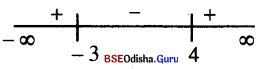⇒ x ∈ [ – 3, 4]
∴ dom f =[-3, 4]

(ix) [x] – x
Solution:
y = f(x) = [x] – x
f(x) is well defined for all x ∈ R
∴ dom f = R

(x) $$\frac{1}{\sqrt{1-x^2}}$$
Solution:
y = f(x) = $$\frac{1}{\sqrt{1-x^2}}$$
f(x) is well defined for 1 – x2 > 0
⇒ x2 < 1 ⇒ – 1 < x < 1
∴ dom f = (-1, 1)

(xi) log (sin x)
Solution:
y = f(x) = log (sin x)
fix) is well-defined for sin x > 0
⇒ x ∈ (2nπ , (2n + l)π), n ∈ Z

Question 4.
Find the range of the following functions:
(i) $$\frac{x^2-1}{x^2+1}$$
Solution:
Let y = f(x) = $$\frac{x^2-1}{x^2+1}$$
⇒ x2 – y + y = x2 – 1
⇒  x2y – x2 = – (1 + y)
⇒ x2 (y- 1)=-(1 + y)
⇒ x2 = $$\frac{\ – (1+y)}{y-1}=\frac{1+y}{1-y}$$
⇒ x = ± $$\sqrt{\frac{1+y}{1-y}}$$
Now x is well-defined for
$$\frac{1+y}{1-y}$$ ≥ 0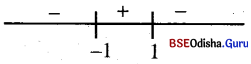∴ rng f = [-1,1)

(ii) $$\sqrt{x-1}$$
Solution:
y = f(x) = $$\sqrt{x-1}$$
⇒ y2 = x- 1
⇒ x = y2 + 1
x is well difierid for all y ∈ R but
y ≥ 0
∴ rng f = [0, ∞]

(iii) [x] – x
Solution:
y = f(x) = [x], – x
We have [x] ≤ x < [ x] + 1
⇒ 0 ≤ x – [x] < 1
⇒ 0 ≥ [x] – x > – 1
⇒ -1 < [x] – x ≤ 0
⇒ -1 < y ≤ 0
⇒ rng f = ( – 1 , 0]

(iv) $$\frac{x}{1-x}$$
Solution:
y = f(x) = $$\frac{x}{1-x}$$
⇒ y – xy = x
⇒ xy + x = y
⇒ x (y + 1) = y
⇒ x = $$\frac{y}{y+1}$$
x is well defined for y ≠ – 1
∴ Rng f = R – {- 1}

(v) $$\frac{x}{1+x^2}$$
Solution:
y = f(x) = $$\frac{x}{1+x^2}$$
⇒ y + x2y = x
⇒ x2y – x + y = 0
⇒ x = $$\frac{1 \pm \sqrt{1-4 y^2}}{2 y}$$
x is well defined for 1 – 4y2 ≥ 0 and y ≠ 0
⇒ y2 ≤ $$\frac{1}{4}$$ and y ≠ 0
⇒ – $$\frac{1}{2}$$ ≤ y ≤ $$\frac{1}{2}$$ and y ≠ 0
but for x = 0 we have y = 0
∴ rng f = $$\left[-\frac{1}{2}, \frac{1}{2}\right]$$

(vi) $$\frac{1}{2-\cos 3 x}$$
Solution:
y = f(x) = $$\frac{1}{2-\cos 3 x}$$
we have – 1 ≤ cos 3x ≤ 1
⇒ 1 ≥ – cos 3x ≥ -1
⇒ – 1 ≤- cos 3x ≤ 1
⇒ 2 – 1 < 2 – cos 3x ≤ 3
⇒ 1 ≤ 2 – cos 3x ≤ 3
⇒ 1 ≥ $$\frac{1}{2-\cos 3 x} \geq \frac{1}{3}$$
⇒ $$\frac{1}{3}$$ ≤ y ≤ 1
∴ rng f =[$$\frac{1}{3}$$, 1]

(vii) log 10 (1 – x)
Solution:
y = f(x) = log10 (1 – x)
Clearly y ∈ R
∴ rng f = R

(viii) $$\sqrt{1+x^2}$$
Solution:
y = f(x) = $$\sqrt{1+x^2}$$
⇒ y2 = 1 + x2
⇒  x2 = y2 – 1
⇒ x = ± $$\sqrt{y^2-1}$$
x is well defined for y2 – 1 ≥ 0
⇒ y2 ≥ 1
⇒ – 1 ≥ y ≥ 1
But y > 0
∴ rng f = [1, ∞)Question 5.
Find the domain and range of the following functions:
(i) $$\frac{x^2}{1+x^2}$$
Solution:
y = f(x) = $$\frac{x^2}{1+x^2}$$
Domain:
f(x) is well defined for all x ∈ R
∴ dom f = R
Range (Method – 1):
y = $$\frac{x^2}{1+x^2}$$
⇒ y + yx2 = x2
⇒ x2 – yx2 = y
⇒ x2 (1 – y) = y
⇒ x2 = $$\frac{y}{1-y}$$
⇒ x = ± $$\sqrt{\frac{y}{1-y}}$$
x is well-defined for $$\frac{y}{1-y}$$ ≥ 0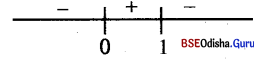⇒ y 6 [0. 1)
∴ Rng f = [0,1)
Method – 2:
0 ≤ x2 < x2 + 1
⇒ 0 ≤ $$\frac{x^2}{x^2+1}$$ < 1
⇒ 0 ≤ y ≤ 1
∴ rng f = [0, 1)

(ii) $$\sqrt{2 x-3}$$
Solution:
y = f(x) = $$\sqrt{2 x-3}$$
Domain:
f(x) is well defined for 2x- 3 ≥ 0
⇒ x ≥ 3/2
∴ dom f =[$$\frac{3}{2}$$, ∞]
Range:
y = $$\sqrt{2 x-3}$$ ⇒ y2 = 2x – 3
⇒ 2x = y2 + 3
⇒ x = $$\frac{y^2+3}{2}$$
x is well defined for all y ∈ R
But y is not negative.
∴ Rng f = [0, ∞)

(iii) loge |x – 2|
Solution:
y = f(x) = log |x – 2|
Domain:
f(x) is well defined for |x – 2| >0
⇒ x ≠ 2
∴ dom f = R – {2}
Range:
y = log |x – 2| ∈ R ⇒ rng f = R

Question 6.
Give an example of a step function on [- 1, 3] = {x ∈ R| – 1 ≤ x ≤ 3}.
Solution:
f(x) = $$\left\{\begin{array}{c} -1,-1 \leq x<0 \\ 0,0 \leq x<1 \\ 1,1 \leq x<2 \\ 2,2 \leq x<3 \\ 3,3 \leq x<4 \end{array}\right\}$$

Question 7.
Let X ={ a, b, c}, Y = {1, 2, 3, 4}
(a) Find out which of the following relations are functions and which are not and why?
Solution:
X = [a, b, c}, Y = {1, 2, 3, 4}
(i) {(a, 1), (a, 2), (b, 3), (b, 4)}
Solution:
{(a, 1), (a, 2), (b, 3), (b, 4)} is not a function as ‘a’ has two images in Y.

(ii) {(a, 2), (b, 3), (c, 4)}
Solution:
{(a, 2), (b, 3), (c, 4)} is a function from X to Y as the elements of X has unique images in Y.

(iii) {(a, 3), (b, 1), (a, 4), (c, 2)}
Solution:
{(a, 3), (b, 1), (a, 4), (c, 2)} is not a function as ‘a’ and ‘b’ have two images also domain of the function ≠ X.

(iv) {(a, 1), (b, 1), (c, 1)}
Solution:
{(a, 1), (b, 1), (c, 1)} is a function from X to Y.

(v) {(a, 2), (b. 1), (c, 1)}
Solution:
{(a, 2), (b, 1), (c, 1)} is a function from X to Y.

(vi) {(a, a), (b, b), (c, c)}
Solution:
{(a, a), (b, b), (c, c)} is a function from X to X.

(b) Find the domain and range of those relations in a which are functions.
Solution:
(i) The domain of each function is
X = [a, b, c}
Range of functions

(ii) is {2, 3, 4}.
In (iv) is {1} and in (v) is {1, 2}. The range of function in (vi) is X.

(c) Identify the constant function if any.
Solution:
The function in (iv)
i.e. {(a, 1), ib, 1), (c, 1)} is a constant function.

(d) Identify the identity function if any.
Solution:
The function in (vi)
i.e.{(a, a), (b, b), (c, c)} is an identity function.

Question 8.
Find $$f(\sqrt{2})$$ and $$\boldsymbol{f}(-\sqrt{3})$$ for the function
$$f(x)=\left\{\begin{array}{l} x^2, \text { if } x<0 \\ x, \text { if } 0 \leq x \leq 1 \\ \frac{1}{x}, \text { if } x>1 \end{array}\right.$$
Solution:
$$f(\sqrt{2})$$ = $$\frac{1}{\sqrt{2}}$$
$$\boldsymbol{f}(-\sqrt{3})$$ = (√3)2 = 3

Question 9.
Find x for which the value of f(x) = x2 – 4x + 3 is
Solution:
f(x) = x2 – 4x + 3
(i) 0
Solution:
f(x) = 0
⇒ x2 – 4x + 3 = 0
⇒ (x – 3) (x – 1) = 0
⇒ x =1, 3

(ii) – 1
Solution:
f(x) = – 1
⇒ x2 – 4x + 3 = -1
⇒ x2 – 4x + 4 = 0
⇒ (x – 2)2 = 0
⇒ x = 2Question 10.
Find the values of x for which the following functions are not defined:
(i) $$\frac{x^2-4}{x-2}$$
Solution:
y = f(x) = $$\frac{x^2-4}{x-2}$$
Clearly f(x) is not defined for x = 2

(ii) $$\frac{\sin x}{x}$$
Solution:
y = f(x) = $$\frac{\sin x}{x}$$
f(x) is not defined for x = 0

(iii) $$\frac{\log \cos x}{\sec x}$$
Solution:
y = f(x) = $$\frac{\log \cos x}{\sec x}$$
f(x) is not defined for cos x ≤ 0
⇒ for x ∈ x $$\left[\frac{\pi}{2}, \frac{3 \pi}{2}\right]$$ or $$\left[\frac{5 \pi}{2}, \frac{7 \pi}{2}\right]$$
In general x ∈ $$\left[(4 n+1) \frac{\pi}{2},(4 n+3) \frac{\pi}{2}\right]$$, n ∈ Z

Question 11.
Let f(x) = $$\sqrt{1+x}$$, g(x) = $$\sqrt{1-x}$$
Find & Also, find the domain in each case.
Solution:
f(x) = $$\sqrt{1+x}$$, g(x) = $$\sqrt{1-x}$$
f(x) is well defined for x + 1 ≥ 0
⇒ x ≥ – 1
∴ dom f = [- 1 , ∞) = D1
g(x) is well defined for 1 – x ≥ 0
⇒ x ≤ 1
⇒ x ∈ (-∞, 1]
∴ dom g (-∞, 1] = D2
∴ D1 ∩ D2 = [-1, 1]
(i) f + g
Solution:
f + g: [-1, 1] → R
defined as (f + g) (x)
= f(x) + g(x) = $$\sqrt{1-x}$$ + $$\sqrt{1-x}$$

(ii) f – g
Solution:
f – g :[-1, 1] → R defined as
(f – g) (x) = $$\sqrt{1-x}$$ – $$\sqrt{1-x}$$

(iii) fg
Solution:
fg : [ – 1, 1] → R defined as
(fg) (x) > f(x) g(x)
$$\sqrt{1-x}$$$$\sqrt{1-x}$$ = $$\sqrt{1-x^2}$$

(iv) f/g
Solution:
f/g : [- 1,1) → R defined as
$$\left(\frac{f}{g}\right)$$(x) = $$\frac{f(x)}{g(x)}=\frac{\sqrt{1+x}}{\sqrt{1-x}}$$
for (i) to (iii) domain = [- 1, 1]
but for (iv) domain = [- 1, 1)

Question 12.
If(x) = loge $$\left(\frac{1-x}{1+x}\right)$$, then prove that f(x) + f(y) = $$\left(\frac{x+y}{1+x y}\right)$$
Solution:
f(x) = log$$\left(\frac{1-x}{1+x}\right)$$
f(x) + f(y) = log$$\left(\frac{1-x}{1+x}\right)$$ + $$\left(\frac{1-y}{1+y}\right)$$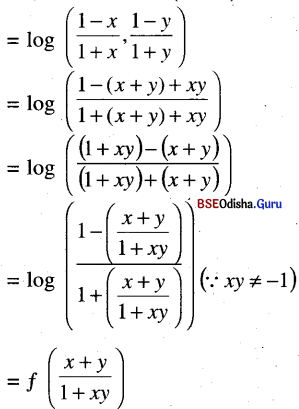Question 13.
Let f = {(-1, 4), (2, 7), (-2, 11}. (0,1), (1, 2) be a quadratic polynomial from Z to Z, find f(x).
Solution:
Let f(x) = ax2 + by2 + C is the quadratic polynomial
f(-1) = 4 ⇒ a – b + c = 4    …..(1)
f(0) = 1⇒ c = 1                   ….(2)
f(1) = 2 ⇒ a + + c = 2        ….(3)
∴ a – b + 1 = 4 and a + b + 1 = 2
⇒ a – b = 3 and a + b = 1
⇒ a = 2, b = -1
f(x) = 2x2 – x + 1
f(2) = 8 – 2 + 1 = 7
f(- 2) = 8 + 2 + 1 = 1 1
Thus the required function is f(x) = 2x2 – x + 1Question 14.
Sketch the graphs of the following functions.
(i) f(x) = x3
(ii) f(x) = 1 + $$\frac{1}{x^2}$$
(iii) f(x) = (x-1)2
Solution:
(i) f(x) = x3

 x 0 1 2 – 1 -2 3 y 1 1 8 -1 -8 27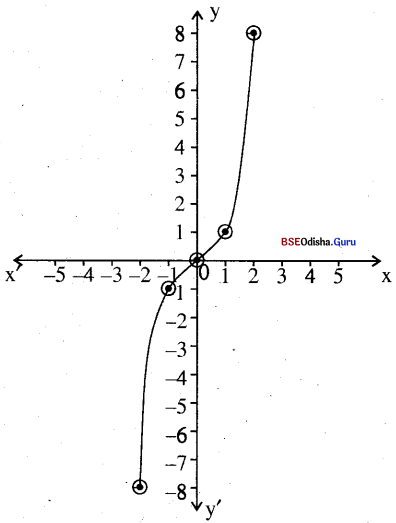(ii) f(x) = 1 + $$\frac{1}{x^2}$$

 x 1 -1 2 – 2 3 -3 y 2 2 5/4 5/4 10/9 10/9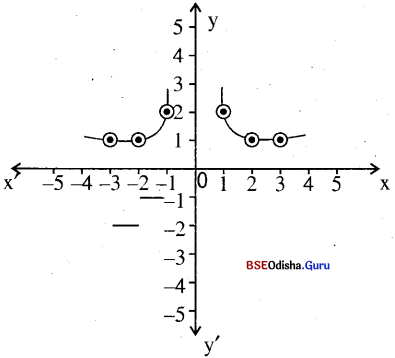(iii) f(x) = (x-1)2

 x -1 0 1 2 3 4 f(x) 4 1 0 1 4 9